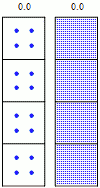#### Learning Objective

• Calculate the half-life of a radioactive element

#### Key Points

• The relationship between time, half-life, and the amount of radionuclide is defined by: $N={N}_{0}{e}^{-\lambda t}$ .
• The relationship between the half-life and the decay constant shows that highly radioactive substances rapidly transform to daughter nuclides, while those that radiate weakly take longer to transform.
• Since the probability of a decay event is constant, scientists can describe the decay process as a constant time period.

#### Term

• half-lifeThe time required for half of the nuclei in a sample of a specific isotope to undergo radioactive decay.

## Decay Rates

Radioactive decay is a random process at the single-atom level; is impossible to predict exactly when a particular atom will decay. However, the chance that a given atom will decay is constant over time. For a large number of atoms, the decay rate for the collection as a whole can be computed from the measured decay constants of the nuclides, or, equivalently, from the half-lives.Radioactive decay simulationA simulation of many identical atoms undergoing radioactive decay, starting with four atoms (left) and 400 atoms (right). The number at the top indicates how many half-lives have elapsed

Given a sample of a particular radionuclide, the half-life is the time taken for half of its atoms to decay. The following equation is used to predict the number of atoms (N) of a a given radioactive sample that remain after a given time (t):

$N={N}_{0}{e}^{-\lambda t}$

In this equation, λ, pronounced “lambda,” is the decay constant, which is the inverse of the mean lifetime, and N0 is the value of N at t=0. The equation indicates that the decay constant λ has units of t-1.

The half-life is related to the decay constant. If you set N = $\frac{N_0}{2}$ and t = t1/2, you obtain the following:

${t}_{1/2}=\frac{ln2}{\lambda}$

This relationship between the half-life and the decay constant shows that highly radioactive substances are quickly spent, while those that radiate weakly endure longer. Half-lives vary widely; the half-life of 209Bi is 1019 years, while unstable nuclides can have half-lives that have been measured as short as 10−23 seconds.

## Example

What is the half-life of element X if it takes 36 days to decay from 50 grams to 12.5 grams?

50 grams to 25 grams is one half-life.

25 grams to 12.5 grams is another half-life.

So, for 50 grams to decay to 12.5 grams, two half-lives, which would take 36 days total, would need to pass. This means each half-life for element X is 18 days.